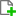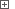# Variables of the Table TypeThis topic describes project, project suite and keyword test variables of the Table type. The topic contains the following sections:

Table variables store two-dimensional arrays. You can use table variables to work with these arrays in tests. These arrays do not correspond to arrays in JavaScript, JScript and VBScript or lists on Python. However, you can use variables of this type to store values of the `SAFEARRAY` type (VBScript-format) arrays. This can be, for example, values returned from various `Find` methods, like `FindAllChildren` or `FindAll`.

The array elements have the Variant type and can store any value: a number, a string, a date and so on. You can also specify the names of array columns and then address the values using these names (see below).

Table variables are not used as permanent data storages. If the contents of such a variable is programmatically initialized and modified at runtime, the changes are not saved after the test execution is over since these variables are intended for temporary usage at runtime. However, it is possible to specify initial values in a table variable before running a test and use the specified data to parameterize tests (for instance, in data-driven tests).

Table variables can be used by the Data-Driven Loop operation to implement the data-driven functionality in keyword tests.

The Keyword Test editor displays keyword test variables of the Table type on the editor’s Test Steps page below the operation tree. You can easily view the variable contents and modify them, if needed.

### Creating Table Variables

You can create Table variables in several ways:

• You create Table variables and set their values by using the Add Variable or Add Variable to Keyword Test wizard or the Variables page of the project, project suite and keyword test editors.

To create a new Table variable, right-click within the page and chooseNew Item from the context menu.

• You can create a Table variable when generating test data with the TestComplete Data Generator wizard. You specify the variable name on the first page of the wizard and on the next page define the table data to be generated.

• You can also create a Table variable when adding the Data-Driven Loop operation to a keyword test or when modifying properties of this operation. You can specify a new variable name on the first page of the operation parameters’ wizard. TestComplete will then display the Data Generator Wizard to let you specify the table contents.

• You can create a Table variable programmatically during the test execution. For this purpose, you can use the `Variables.AddVariable` method. To add columns to a Table variable, use the `AddColumn` method of the `TableVariable` object. To modify the number of rows in the table programmatically, simply change the value of the `RowCount` property.

Below is sample code that demonstrates how to create and initialize a table variable from scripts.

###### ExampleView description

JavaScript

function TableVarTest()
{
// Create a table variable if it doesn't exist
if(!Project.Variables.VariableExists("MyTable"))

// Get a reference to the variable
var t = Project.Variables.VariableByName("MyTable");

// Add columns to the table

// Create two rows
t.RowCount = 2;

// Fill in the table
// The first row
t.\$set("Name", 0, "John Smith");
t.\$set("Age", 0, 24);
t.\$set("City", 0, "Seattle");

// The second row (using the Item property)
t.\$set("Item", "Name", 1, "Bob Feather");
t.\$set("Item", "Age", 1, 59);
t.\$set("Item", "City", 1, "Milltown");
}

JScript

function TableVarTest()
{
// Create a table variable if it doesn't exist
if(!Project.Variables.VariableExists("MyTable"))

// Get a reference to the variable
var t = Project.Variables.VariableByName("MyTable");

// Add columns to the table

// Create two rows
t.RowCount = 2;

// Fill in the table
// The first row
t.Name(0) = "John Smith";
t.Age(0) = 24;
t.City(0) = "Seattle";

// The second row (using the Item property)
t.Item("Name", 1) = "Bob Feather";
t.Item("Age", 1) = 59;
t.Item("City", 1) = "Milltown";
}

Python

``````def TableVarTest():
# Create a table variable if it doesn't exist
if not Project.Variables.VariableExists("MyTable"):

# Get a reference to the variable
t = Project.Variables.VariableByName["MyTable"]

# Add columns to the table

# Create two rows
t.RowCount = 2

# Fill in the table
# The first row
t.Name = "John Smith"
t.Age = 24
t.City = "Seattle"

# The second row (using the Item property)
t.Item["Name", 1] = "Bob Feather"
t.Item["Age", 1] = 59
t.Item["City", 1] = "Milltown"``````

VBScript

Sub TableVarTest
Dim t
' Create a table variable if it doesn't exist
If Not Project.Variables.VariableExists("MyTable") Then
End If

' Get a reference to the variable
Set t = Project.Variables.VariableByName("MyTable")

' Add columns to the table

' Create two rows
t.RowCount = 2

' Fill in the table
' The first row
t.Name(0) = "John Smith"
t.Age(0) = 24
t.City(0) = "Seattle"

' The second row (using the Item property)
t.Item("Name", 1) = "Bob Feather"
t.Item("Age", 1) = 59
t.Item("City", 1) = "Milltown"
End Sub

DelphiScript

procedure TableVarTest;
var t: OleVariant;
begin
// Create a table variable if it doesn't exist
if not Project.Variables.VariableExists('MyTable') then

// Get a reference to the variable
t := Project.Variables.VariableByName('MyTable');

// Add columns to the table

// Create two rows
t.RowCount := 2;

// Fill in the table
// The first row
t.Name(0) := 'John Smith';
t.Age(0) := 24;
t.City(0) := 'Seattle';

// The second row (using the Item property)
t.Item('Name', 1) := 'Bob Feather';
t.Item('Age', 1) := 59;
t.Item('City', 1) := 'Milltown';
end;

C++Script, C#Script

function TableVarTest()
{
// Create a table variable if it doesn't exist
if(!Project["Variables"]["VariableExists"]("MyTable"))

// Get a reference to the variable
var t = Project["Variables"]["VariableByName"]("MyTable");

// Add columns to the table

// Create two rows
t["RowCount"] = 2;

// Fill in the table
// The first row
t["Name"](0) = "John Smith";
t["Age"](0) = 24;
t["City"](0) = "Seattle";

// The second row (using the Item property)
t["Item"]("Name", 1) = "Bob Feather";
t["Item"]("Age", 1) = 59;
t["Item"]("City", 1) = "Milltown";
}

### Editing Table Variables

You modify Table variables on the Variables page of the project, project suite and keyword test editors. To modify a variable, click the ellipsis button in the Default Value column. This invokes the Edit Table Variable dialog. It lets you change the dimension of the array and specify the values of its elements. For detailed information on working with the dialog, see Working With the Table Variable Editor.

Table variables that belong to keyword tests can be changed from the keyword test editor. The editor displays Table variables on special tabbed pages below the operation tree. You can modify the table data directly on these pages. To do this, simply click the desired cell and then type the desired value in the ensuing in-place editor. Press Enter to confirm the change or Esc to cancel it.

If you want to add or remove columns or to add new rows to the table, use the special edit dialog. To invoke it, activate the tabbed page that corresponds to the desired Table variable, click the down-arrow button on the right and choose Edit from the subsequent menu. For information on the actions available in the dialog, see Working With the Table Variable Editor.

### Working With Table Variables

To access Table type variables, use the `Variables` property of the project, project suite or keyword test scripting object. This property provides a scripting interface to the test’s variables. To address a variable, you can use the following syntax:

Project.Variables.Variable_Name
ProjectSuite.Variables.Variable_Name
KeywordTests.Test_Name.Variables.Variable_Name
-- or --
Project.Variables.VariableByName(Variable_Name)
ProjectSuite.Variables.VariableByName(Variable_Name)
KeywordTests.Test_Name.Variables.Variable_Name

To access the desired element of the array, you can use the `Item` property or additional properties of the `TableVariable` object. TestComplete adds them when you are specifying the names of the array columns from the Variables page. These properties are named as columns and have the Index parameter. You use this parameter to specify the row that contains the desired element. Suppose, we have created the MyVar project variable of the Table type and named the first column of the array as CustomerName. The following code snippet demonstrates how you can get the first element from the first column of the array stored in this variable:

JavaScript, JScript

var s;
s = Project.Variables.MyVar.Item(0, 0);
// -- or --
s = Project.Variables.VariableByName("MyVar").CustomerName(0);

Python

``````s = Project.Variables.MyVar.Item[0, 0]
# -- or --
s = Project.Variables.VariableByName["MyVar"].CustomerName``````

VBScript

s = Project.Variables.MyVar.Item(0, 0)
' -- or --
s = Project.Variables.VariableByName("MyVar").CustomerName(0)

DelphiScript

var
s : OleVariant;
begin
s := Project.Variables.MyVar.Item(0, 0);
// -- or --
s := Project.Variables.VariableByName('MyVar').CustomerName(0);
end;

C++Script, C#Script

var s;
s = Project["Variables"]["MyVar"].Item(0, 0);
// -- or --
s = Project["Variables"]["VariableByName"]("MyVar").CustomerName(0);

You can also use the `Item` property to modify the values of the desired Table variables (see the example below).

JavaScript

let s;
s = "John";
Project.Variables.MyVar.\$set("Item", 0, 0, s);
// -- or --
Project.Variables.VariableByName("MyVar").\$set("CustomerName", 0, s);

JScript

var s;
s = "John";
Project.Variables.MyVar.Item(0, 0) = s;
// -- or --
Project.Variables.VariableByName("MyVar").CustomerName(0) = s;

Python

``````s = "John"
Project.Variables.MyVar.Item[0, 0] = s
# -- or --
Project.Variables.VariableByName["MyVar"].CustomerName = s``````

VBScript

s = "John"
Project.Variables.MyVar.Item(0, 0) = s
' -- or --
Project.Variables.VariableByName("MyVar").CustomerName(0) = s

DelphiScript

var
s : OleVariant;
begin
s := 'John';
Project.Variables.MyVar.Item(0, 0) := s;
// -- or --
Project.Variables.VariableByName('MyVar').CustomerName(0) := s;
end;

C++Script, C#Script

var s;
s = "John";
Project["Variables"]["MyVar"].Item(0, 0) = s;
// -- or --
Project["Variables"]["VariableByName"]("MyVar").CustomerName(0) = s;

You can also use Table variables to specify parameters of keyword test operations. You do this in the Operation Parameters dialog that is used to view and modify the operation parameters. For detailed information about this, see Specifying Operation Parameters.

To assign a value to an element of the array stored in the Table type variable from a keyword test, use the Set Variable Value operation. In the Operation Parameters dialog you can specify the variable, the index of the desired element and the value to be assigned.